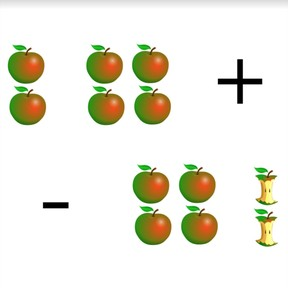Recognizing addition and subtraction situations to 10

# Recognizing addition and subtraction situations to 108,000 schools use Gynzy92,000 teachers use Gynzy1,600,000 students use Gynzy

## General

Students learn to recognize addition or subtraction situations by using an image.

1.OA.A.1

## Relevance

Discuss with students that it is useful to be able to recognize if something is being added or taken away to a situation. You learn how math problems are formed and can learn to add and subtract.

## Introduction

Show the images on the interactive whiteboard and ask students to determine which one shows 9 watering cans. Tell students that they can cross off the objects as they count them, to make counting easier. Then show students the water droplets and ask them to determine where they see 5 water droplets.

## Development

Using the glasses of lemonade shown on the interactive whiteboard, show the students an 'adding to' situation. There are glasses that have already been filled, and glasses that still need to be filled. First count the filled glasses and then the empty glasses. There is 1 glass with lemonade, and 7 glasses without lemonade. The glasses without lemonade will be added to the 1 full glass (as they will all be filled) so the math problem is 1 + 7. Discuss all the variations with the lemonade and show the accompanying math problem to each image.
Then show students a 'taking away' situation. Use the glasses of lemonade again, and again, show filled glasses and empty glasses. First count how many glasses there are in total, and then count how many glasses are empty. There are 10 glasses, and 3 are empty. If you take away the empty glasses from all of the glasses, you have 7 glasses left that have lemonade. The glasses that are empty have been drunk and are taken away. The math problem is 10 - 7. Discuss all the variations with the lemonade and show the accompanying math problem to each image.
Next discuss how to find the math problem using the cake with strawberries. You can see that the hands are adding more strawberries to the cake. Determine the problem by first counting how many strawberries are on the cake, which is the first number of the addition problem, then counting how many strawberries are in the hands (waiting to be added), which is the second number of the addition problem, and then counting those two numbers together. Discuss a situation in which things are taken away using the fish in the aquarium. The first number is the total number of fish in the aquarium. The second number is the number of fish you take out of the aquarium. Practice more adding to situations with the bead chain and taking away problems with the chocolates.
Tell students that now that you can determine what math problem is being represented by an image, you can also find the total or difference. Using the cake, show students that you can solve an adding to problem by counting the numbers together to find the total. Using the aquarium, show students that you can solve a taking away problem by taking away the second number from the first to find the difference. To practice both addition and subtraction, show students a set of adding to and taking from problems to solve. Ask students to first determine what the math problem is, and then to solve. Have students explain their strategy.

Check that students are able to recognize addition or subtraction problems using an image by asking the following questions:
- How do you know which numbers to use in a math problem?
- What do you do when you want to find the total of a set of numbers?
- What do you do if you want to find the difference between a set of numbers?

## Guided practice

Students are first given problems in which they are asked to recognize or write the math problem that matches the given image. They are then asked to write and solve the math problem represented by the given image.

## Closing

Remind students that it is important to be able to recognize if a situation is addition or subtraction so that they can learn to determine how many they have, or have left. Ask students to form groups. In groups, they must determine what the math problems are for the set of images given. When they have written down the math problems and solved them, all the students in the group must stand up. Which group is quickest? You may choose to ask students to write the math problems and solve them, or to simply write the problems and then to solve them as a class.

## Teaching tips

Students who have difficulty adding or subtracting using an image can be supported by the use of MAB blocks or other manipulatives. To practice addition, have them make two groups of blocks, one for each number. Then count the blocks on the left, and use the blocks on the right to count on. To practice subtraction, have them make one group of blocks for the first number. Then they should take away one block at a time until they create a pile that is equal to the second number. The amount left is the difference.

## Instruction materials

Optional: MAB blocks or other manipulatives.

### The online teaching platform for interactive whiteboards and displays in schools

• Save time building lessons

• Manage the classroom more efficiently

• Increase student engagement